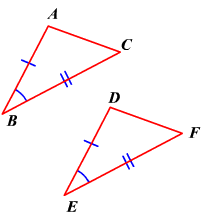# SAS (Side-Angle-Side) Postulate

If two sides and the included angle of a triangle are congruent to two sides and the included angle of another triangle, then the two triangles are congruent.In the figure, $\stackrel{¯}{AB}\cong \stackrel{¯}{DE},\stackrel{¯}{BC}\cong \stackrel{¯}{EF},\text{\hspace{0.17em}}\text{\hspace{0.17em}}\text{and}\text{\hspace{0.17em}}\text{\hspace{0.17em}}\angle B\cong \angle E$ .

Therefore, by the SAS postulate, $\Delta ABC\cong \Delta DEF$ .